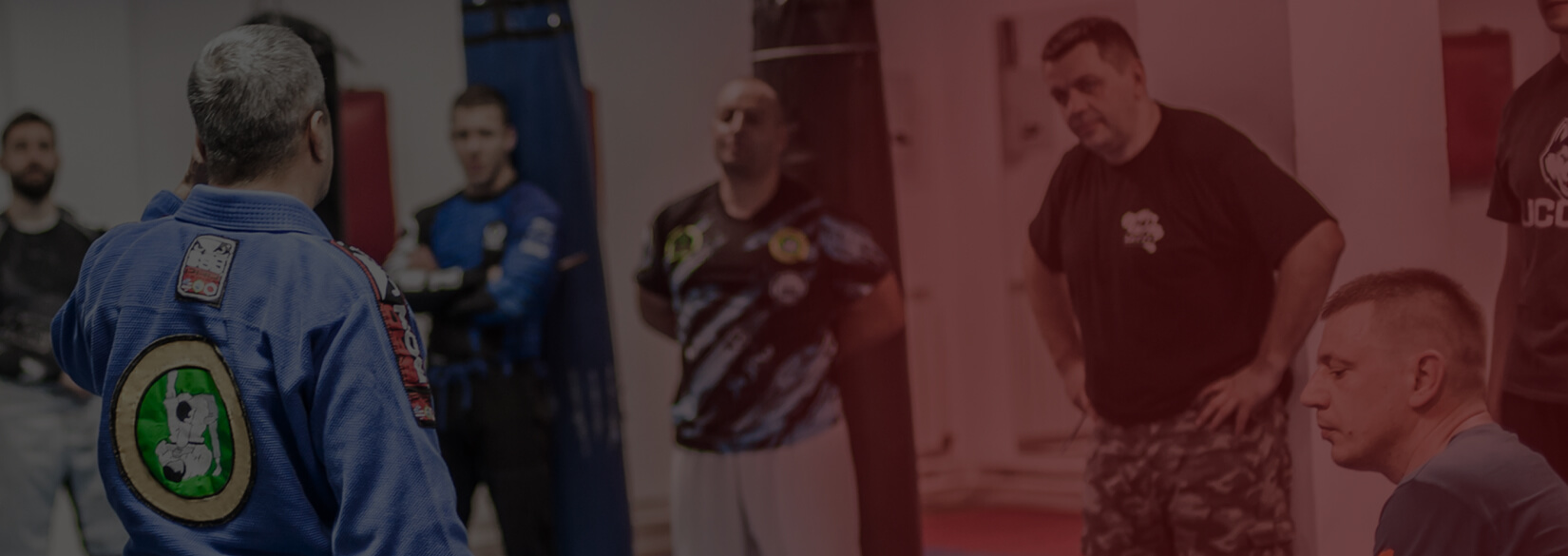# Case Study

## Jiujitsu PayFull Service Payment Processing For Jiujitsu Gyms. Jiujitsu Payments is the simple, affordable way for Jiujitsu Gyms to accept payments.## Problem

Here is where you add the problems. Here is where you add the problems. Here is where you add the problems. Here is where you add the problems. Here is where you add the problems. Here is where you add the problems. Here is where you add the problems.## Solution

Here is where you add the problems. Here is where you add the problems. Here is where you add the problems. Here is where you add the problems. Here is where you add the problems. Here is where you add the problems. Here is where you add the problems.## Outcome

Here is where you add the problems. Here is where you add the problems. Here is where you add the problems. Here is where you add the problems. Here is where you add the problems. Here is where you add the problems. Here is where you add the problems.## Website Design and Development

You can add the contents for this project here.

### Logo

You can add the contents for this project here. You can add the contents for this project here. You can add the contents for this project here. You can add the contents for this project here. You can add the contents for this project here.